Ex 1.2

Chapter 1 Class 7 Integers
Serial order wise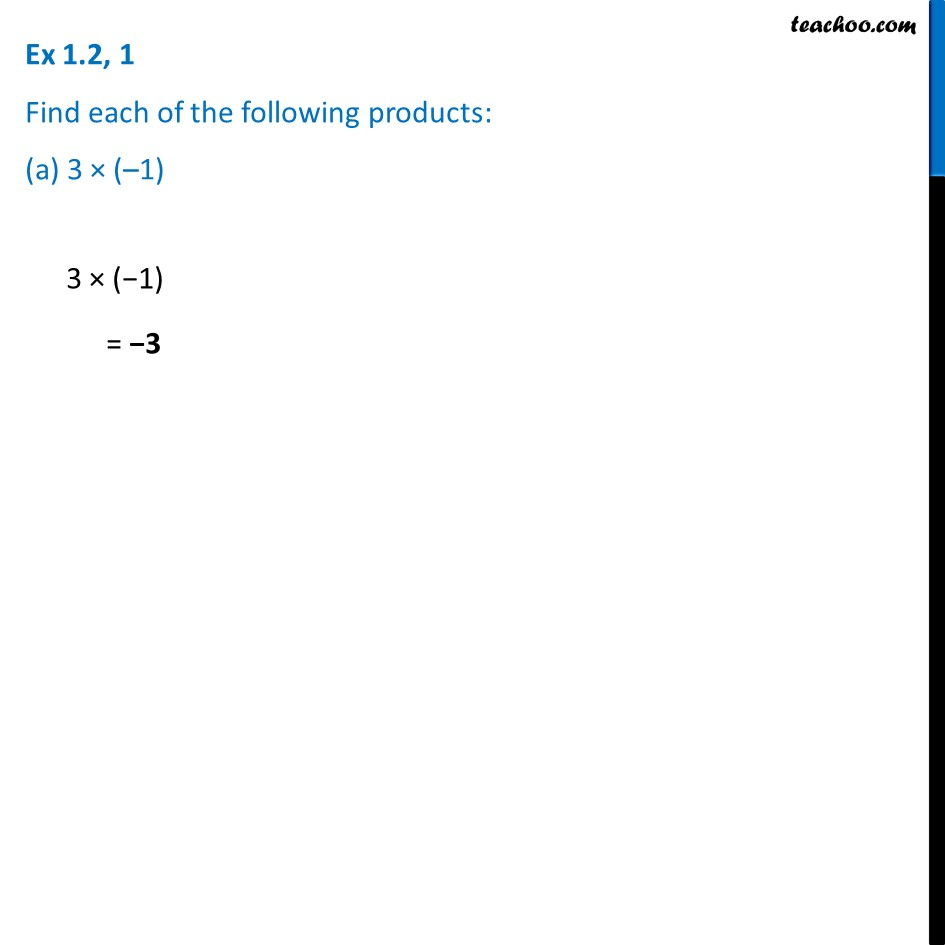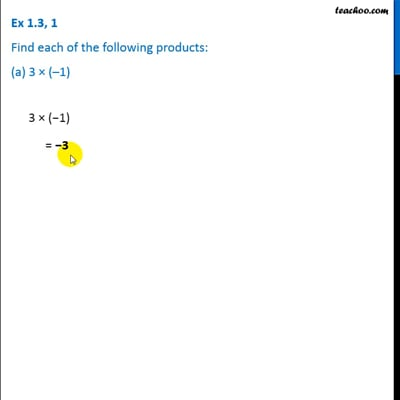This video is only available for Teachoo black users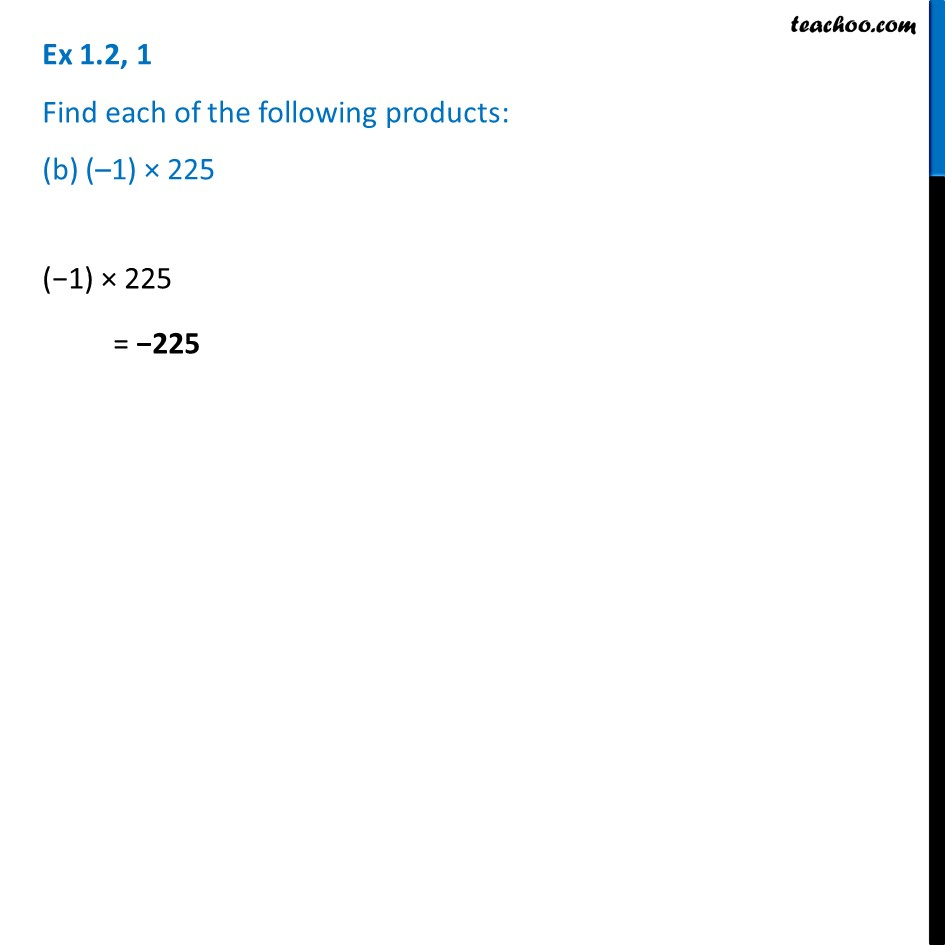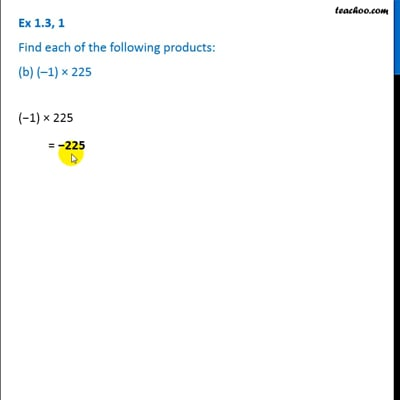This video is only available for Teachoo black users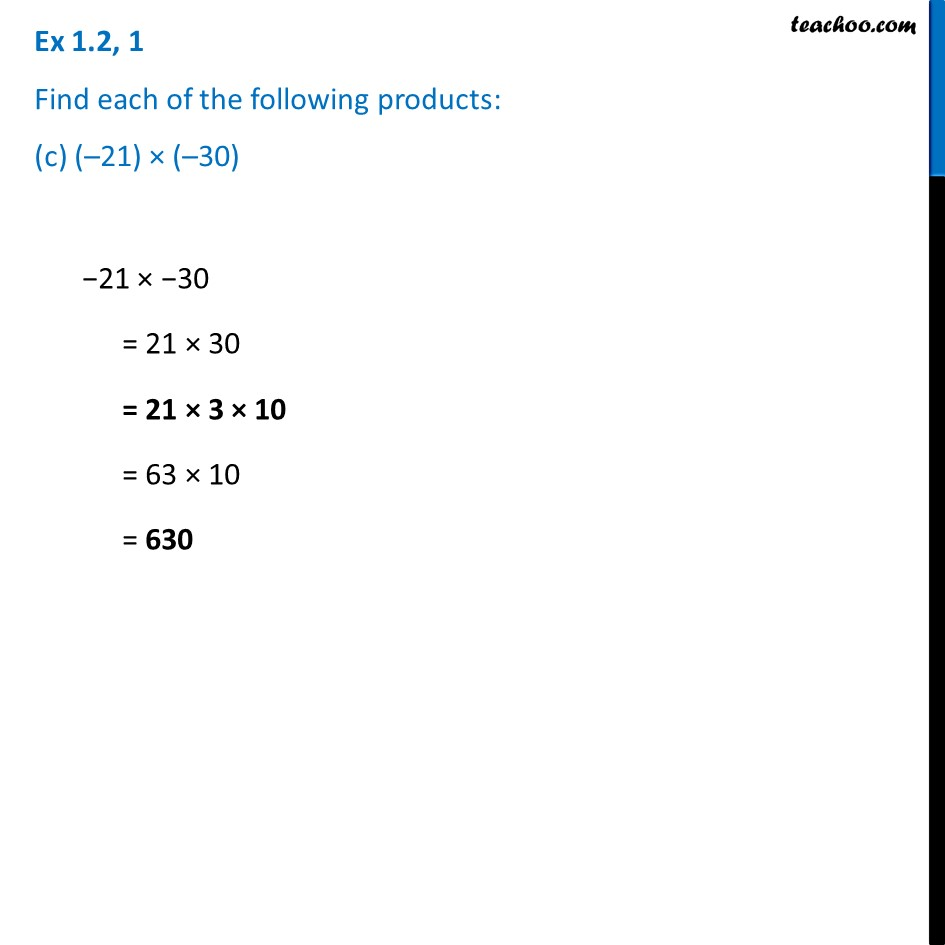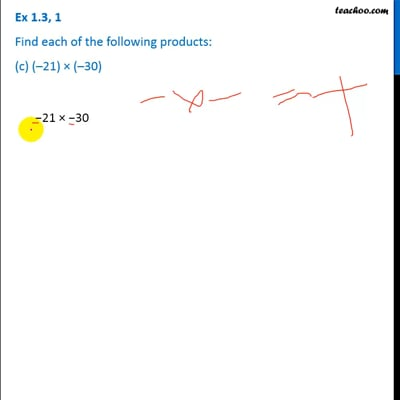This video is only available for Teachoo black users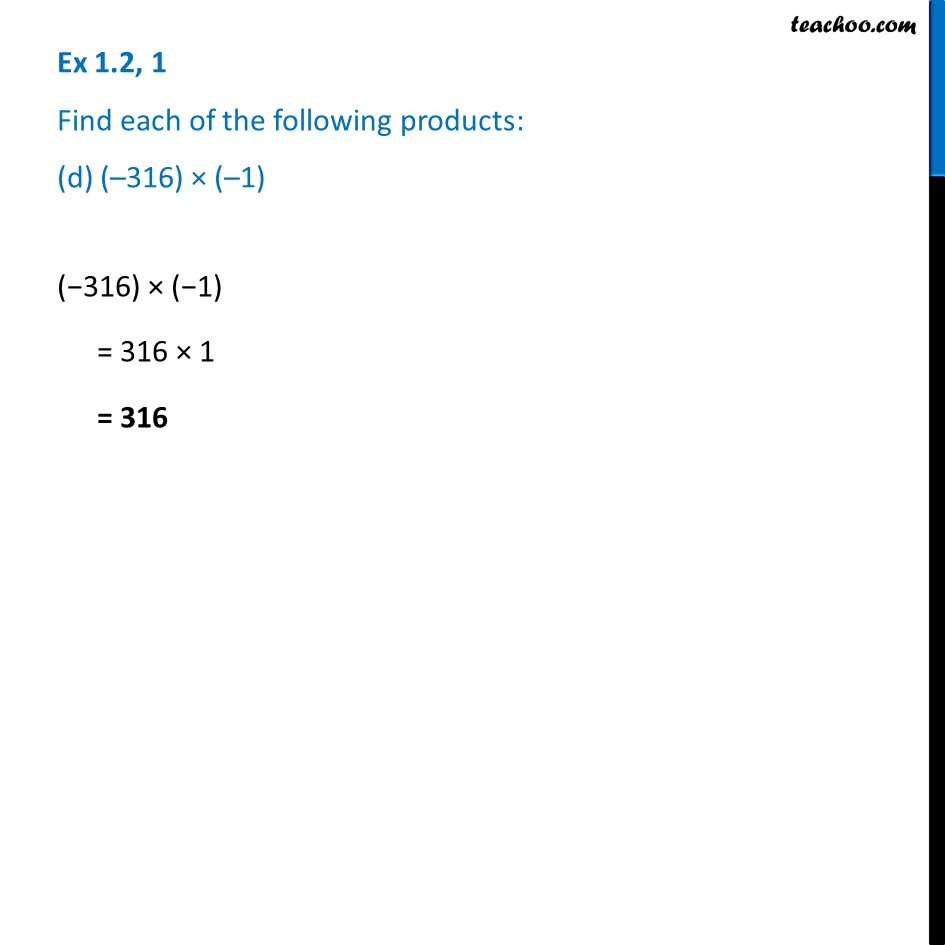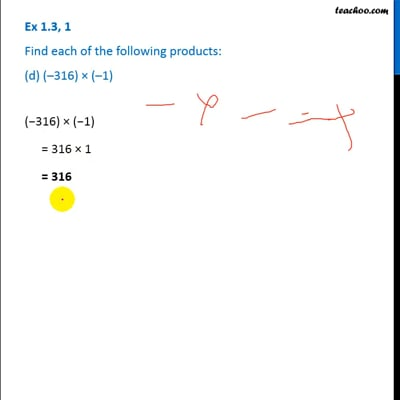This video is only available for Teachoo black users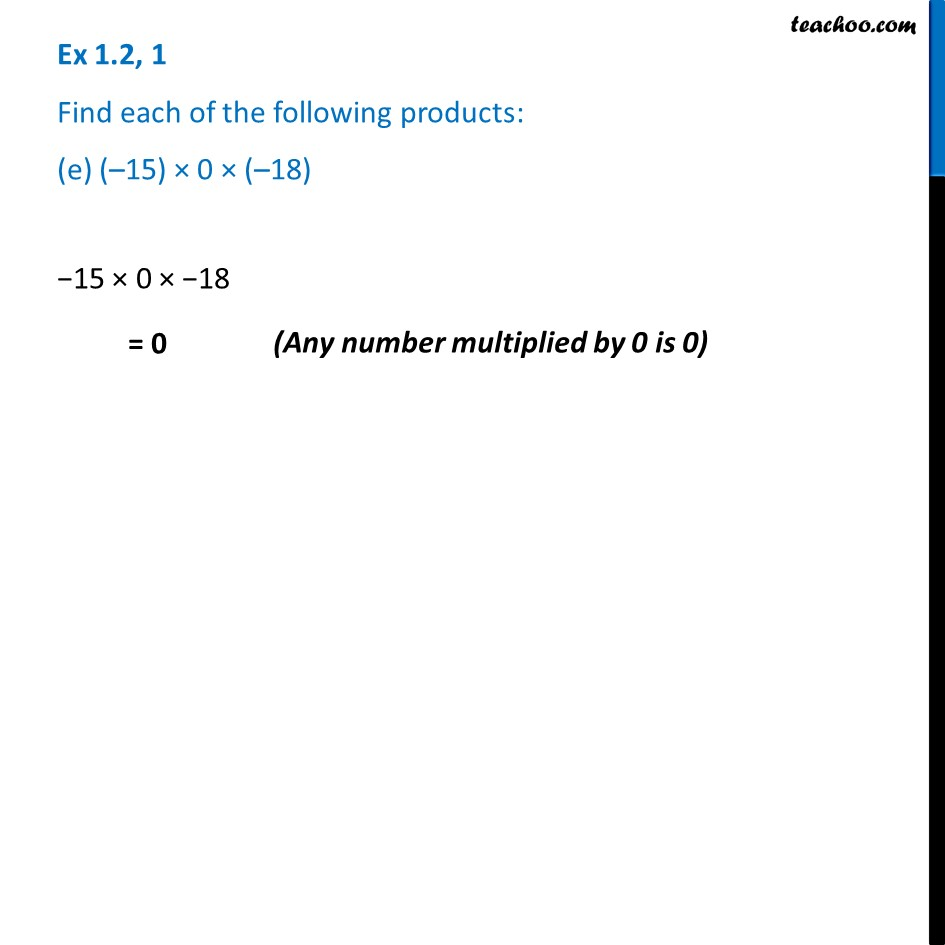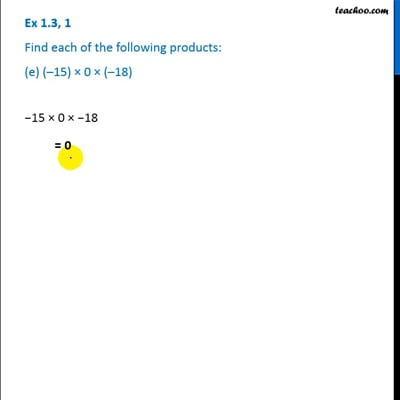This video is only available for Teachoo black users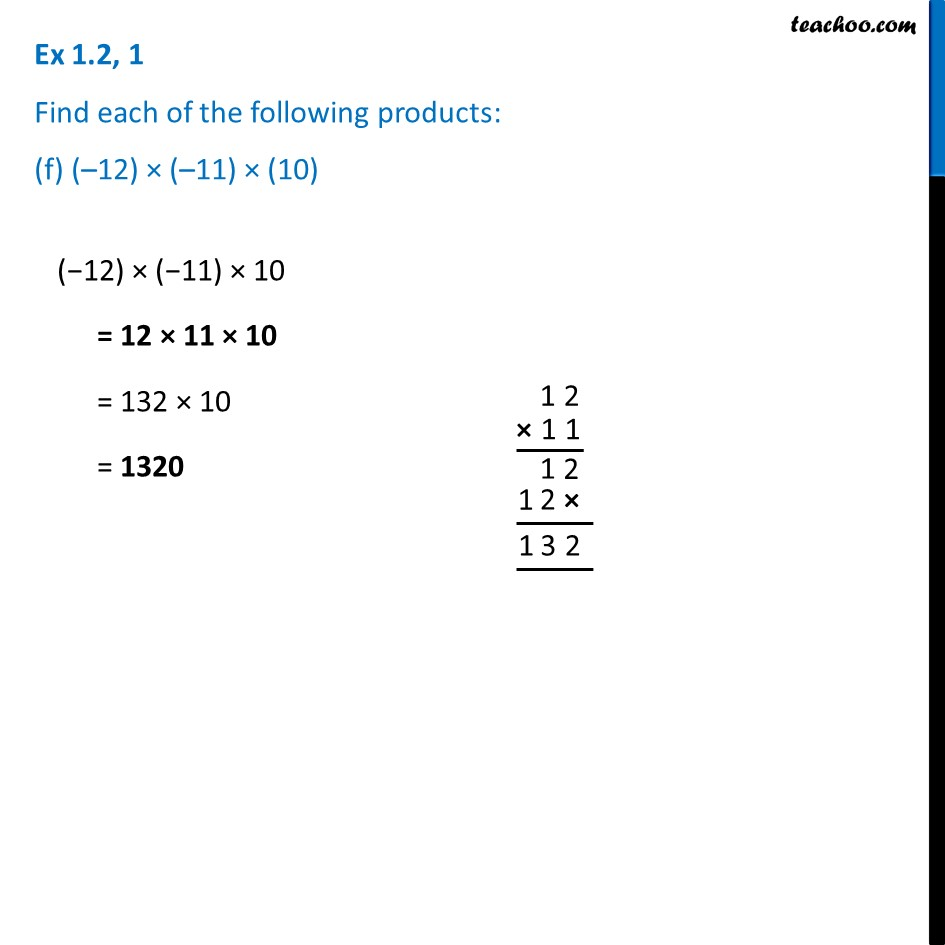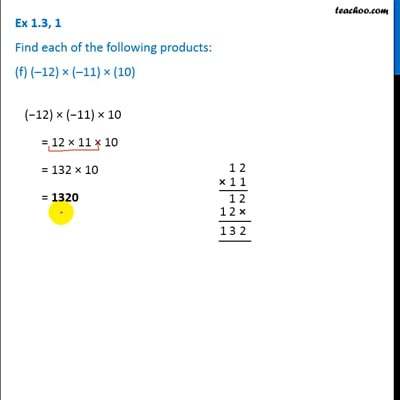This video is only available for Teachoo black users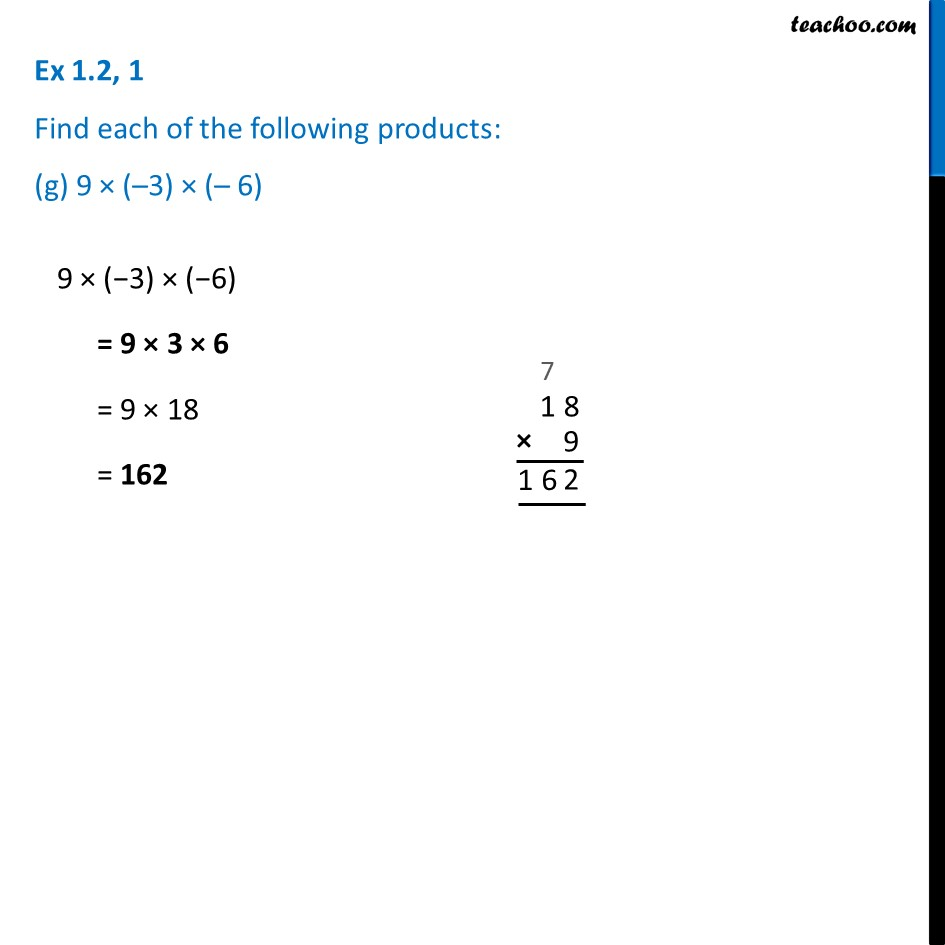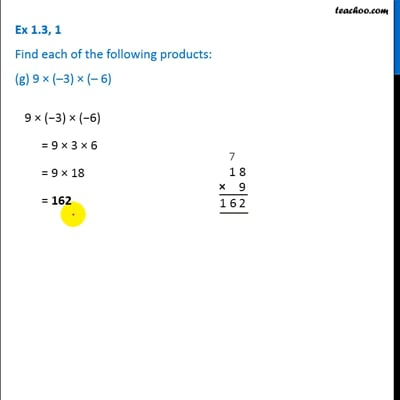This video is only available for Teachoo black users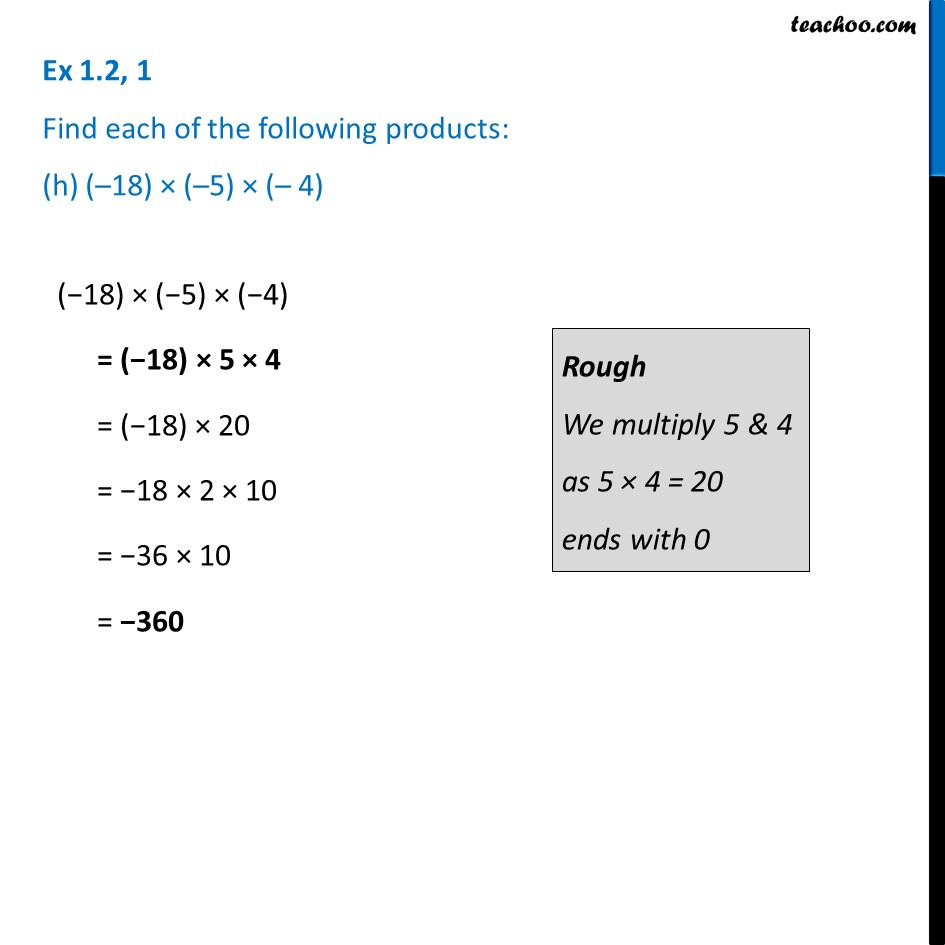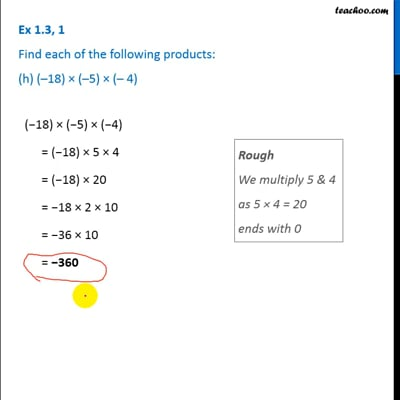This video is only available for Teachoo black users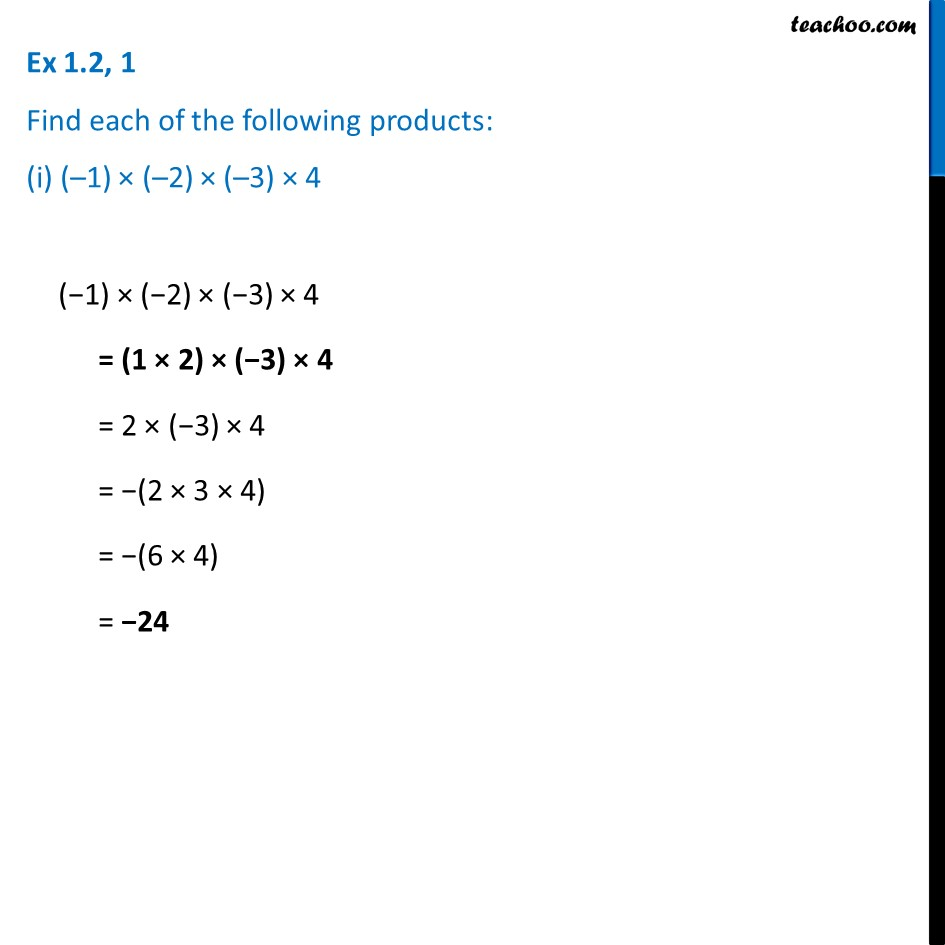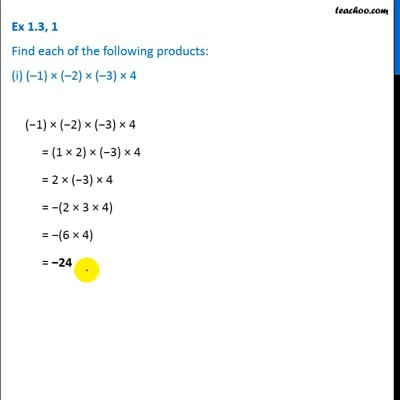This video is only available for Teachoo black users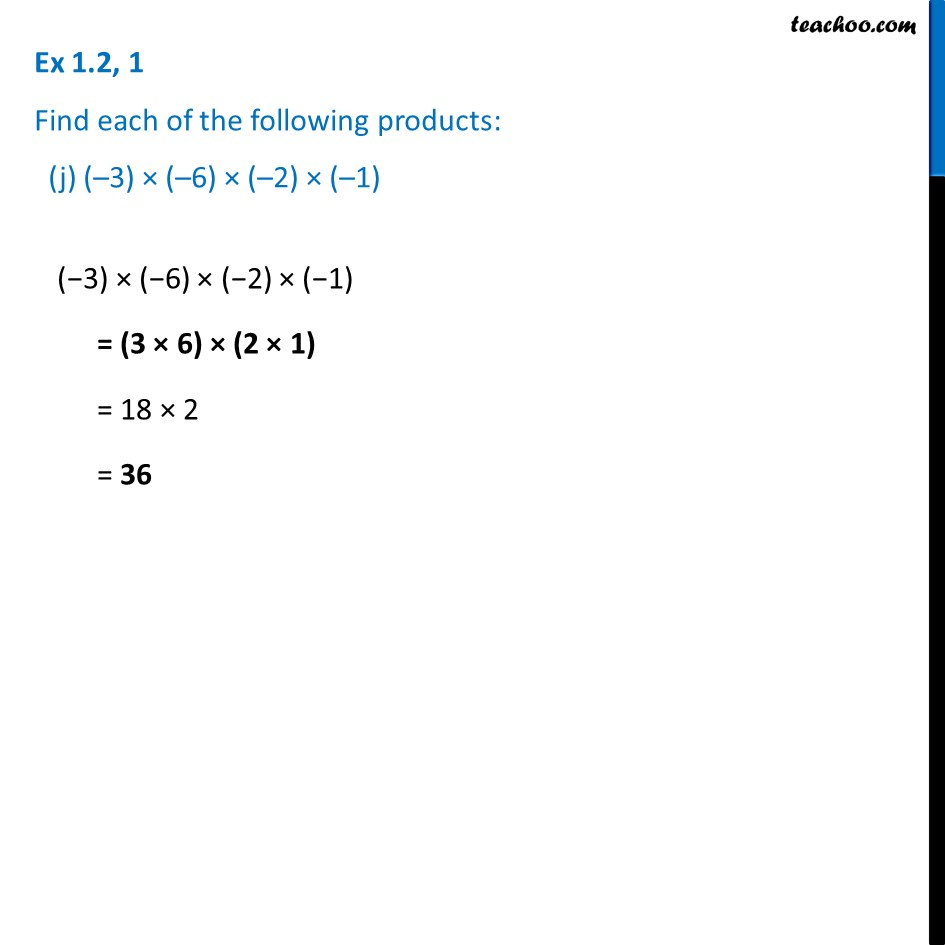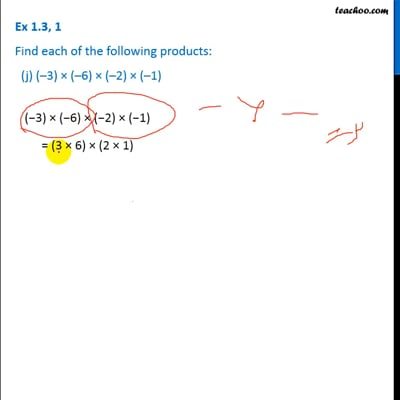This video is only available for Teachoo black users

Learn in your speed, with individual attention - Teachoo Maths 1-on-1 Class

### Transcript

Ex 1.2, 1 Find each of the following products: (a) 3 × (–1) 3 × (−1) = −3 Ex 1.2, 1 Find each of the following products: (b) (–1) × 225 (−1) × 225 = −225 Ex 1.2, 1 Find each of the following products: (c) (–21) × (–30) −21 × −30 = 21 × 30 = 21 × 3 × 10 = 63 × 10 = 630 Ex 1.2, 1 Find each of the following products: (d) (–316) × (–1) (−316) × (−1) = 316 × 1 = 316 Ex 1.2, 1 Find each of the following products: (e) (–15) × 0 × (–18) −15 × 0 × −18 = 0 Ex 1.2, 1 Find each of the following products: (f) (–12) × (–11) × (10) (−12) × (−11) × 10 = 12 × 11 × 10 = 132 × 10 = 1320 Ex 1.2, 1 Find each of the following products: (g) 9 × (–3) × (– 6) 9 × (−3) × (−6) = 9 × 3 × 6 = 9 × 18 = 162 Ex 1.2, 1 Find each of the following products: (h) (–18) × (–5) × (– 4) (−18) × (−5) × (−4) = (−18) × 5 × 4 = (−18) × 20 = −18 × 2 × 10 = −36 × 10 = −360 Ex 1.2, 1 Find each of the following products: (i) (–1) × (–2) × (–3) × 4 (−1) × (−2) × (−3) × 4 = (1 × 2) × (−3) × 4 = 2 × (−3) × 4 = −(2 × 3 × 4) = −(6 × 4) = −24 Ex 1.2, 1 Find each of the following products: (j) (–3) × (–6) × (–2) × (–1)(−3) × (−6) × (−2) × (−1) = (3 × 6) × (2 × 1) = 18 × 2 = 36